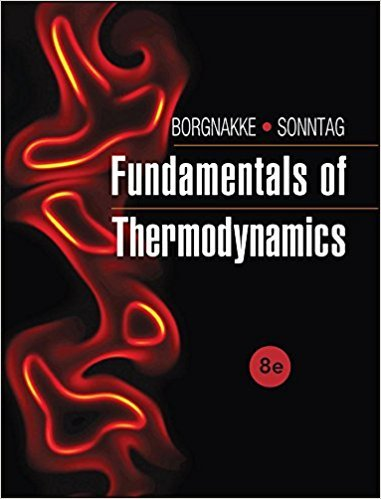×
Get Full Access to Fundamentals Of Thermodynamcs - 8 Edition - Chapter 3 - Problem 226hp
Get Full Access to Fundamentals Of Thermodynamcs - 8 Edition - Chapter 3 - Problem 226hp

×

# A piston held by a pin in an insulated cylinder, shown inISBN: 9781118131992 58

## Solution for problem 226HP Chapter 3

Fundamentals of Thermodynamcs | 8th Edition

• Textbook Solutions
• 2901 Step-by-step solutions solved by professors and subject experts
• Get 24/7 help from StudySoup virtual teaching assistantsFundamentals of Thermodynamcs | 8th Edition

4 5 1 287 Reviews
23
3
Problem 226HP

Problem 226HP

A piston held by a pin in an insulated cylinder, shown in Fig. P3.226, contains 2 kg of water at 100°C, with a quality of 98%. The piston has a mass of 102 kg, with cross-sectional area of 100 cm2, and the ambient pressure is 100 kPa. The pin is released, which allows the piston to move. Determine the final state of the water, assuming the process to be adiabatic.

FIGURE P3.226Step-by-Step Solution:

Solution 226 HP:

Step 1 of 3:-

Here we have to determine the final state of water.

The mass of the water was,.

The temperature was,.

The quality was 98%.

The piston mass is,.

The cross sectional area is,.

The ambient pressure is,.

Step 2 of 3

Step 3 of 3

##### ISBN: 9781118131992

This full solution covers the following key subjects: piston, Water, pin, insulated, assuming. This expansive textbook survival guide covers 7 chapters, and 1462 solutions. The answer to “A piston held by a pin in an insulated cylinder, shown in Fig. P3.226, contains 2 kg of water at 100°C, with a quality of 98%. The piston has a mass of 102 kg, with cross-sectional area of 100 cm2, and the ambient pressure is 100 kPa. The pin is released, which allows the piston to move. Determine the final state of the water, assuming the process to be adiabatic.FIGURE P3.226” is broken down into a number of easy to follow steps, and 71 words. Fundamentals of Thermodynamcs was written by and is associated to the ISBN: 9781118131992. The full step-by-step solution to problem: 226HP from chapter: 3 was answered by , our top Engineering and Tech solution expert on 08/03/17, 05:05AM. Since the solution to 226HP from 3 chapter was answered, more than 412 students have viewed the full step-by-step answer. This textbook survival guide was created for the textbook: Fundamentals of Thermodynamcs , edition: 8.

Unlock Textbook Solution